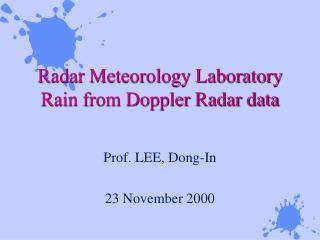Download PresentationRadar Meteorology Laboratory Rain from Doppler Radar data - PowerPoint PPT Presentation

Radar Meteorology Laboratory Rain from Doppler Radar data. Prof. LEE, Dong-In 23 November 2000. Object: To get rainfall from radar data. Theory:. Add appropriate theory from text or other sources. Radar reflectivity factor.I am the owner, or an agent authorized to act on behalf of the owner, of the copyrighted work described.
Download PresentationDownload Policy: Content on the Website is provided to you AS IS for your information and personal use and may not be sold / licensed / shared on other websites without getting consent from its author.While downloading, if for some reason you are not able to download a presentation, the publisher may have deleted the file from their server.

- - - - - - - - - - - - - - - - - - - - - - - - - - E N D - - - - - - - - - - - - - - - - - - - - - - - - - -
Presentation Transcript
1. Radar Meteorology LaboratoryRain from Doppler Radar data Prof. LEE, Dong-In 23 November 2000

2. Object: To get rainfall from radar data

3. Theory: • Add appropriate theory from text or other sources.

4. Radar reflectivity factor where z is radar reflectivity factor (mm6/m3), Ni is the number of drops per unit volume (m-3) and Di is diameter (mm) for drops in the ith size category.

5. Rain Rate • Rain rate is the rate at which water volume accumulates over a unit area and is given by where A is the area involved and t is time. • This equation actually calculates the volume of water per unit area per unit time.

6. Liquid Water Content • Liquid water content is the mass of liquid water in a volume in space, given by where viis the terminal velocity of the ith diameter raindrop, and rw is the density of water.

7. Z-R Relationship

8. Procedure: • Get Radar data during rain situation • Trace contours of reflectivity from each time onto a single image. • “Connect the dots” and draw swaths of the path of the storm at each reflectivity level. • Convert Z to R • Get rain at each time by integrating amount over each location at each time.

9. (Ex.) NEXRAD images of rain • Get data at hourly intervals. • One possible way is to get it from: • http://wxp.atms.purdue.edu/radar/previous/rad_comp-X.gif where X can be 1, 2, 3, 4, 5, or 6. • Alternatively, get more detailed data from a specific site.

10. We can combine these into a single image.

11. You may want to select a single region for your analysis.

12. Transfer Z data from each image to a common image. • Draw outlines of each reflectivity level onto a single piece of acetate or a separate image. • Then combine these.

13. Now combine individual images into a single image.

14. “Connect the dots” to produce rain swaths.

15. Fill the contours if you like.

16. Convert Z to R • Use Z-R relationship. • z = 300 R1.5 where R is rainrate in mm/h and z is radar reflectivity factor in mm6/m3

17. Razzelfratz!!! • After spending an hour or so generating all the (ugly) images before this, it finally dawned on me that this is not necessarily going to answer the question: “How much did it rain at point X?” • How can we answer that question?

18. Questions: • Define “rainfall” • How do we go from radar data to rainfall accumulations? • What points (on the ground) are good ones to use? • How much time resolution in the radar data is needed? Does it depend on the kind of rain falling?

19. Rainfall total where R is the rainrate (mm/h) at a given place and time, Dt is the time interval between consecutive radar data sets. Rtotal = the total depth of rain at the location over the entire time interval.

20. How do we get R? • Radar give radar reflectivity factor Z (or z). • From a z-R relationship, we can convert z into R. • z = ARbwhere A and b are empirical constants, R is rainrate in mm/h, and z is the linear radar reflectivity factor in mm6/m3. • Use: z = 200R1.6, the Marshall-Palmer z-R relationship.